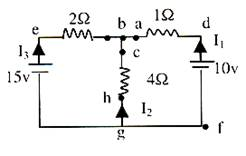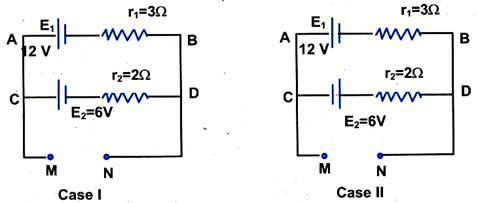Click to Chat

1800-1023-196

+91-120-4616500

CART 0

• 0

MY CART (5)

Use Coupon: CART20 and get 20% off on all online Study Material

ITEM
DETAILS
MRP
DISCOUNT
FINAL PRICE
Total Price: Rs.

There are no items in this cart.
Continue Shopping• Complete Physics Course - Class 11
• OFFERED PRICE: Rs. 2,968
• View Details

```Solved Examples of Comlicated Circuits

Illustration:

Let us analyse a simple circuit shown in the figure alongside. Assume current values (I1, I2 & I3) at random directions.Alt txt:  simple circuit

Solutions

Þ     All through the branch gfdab current in I1

All through the branch geb current is I3

All through the branch ghcb current is I2

Applying KCL at b Þ I1 + I2 + I3 = 0                       …… (i)

(Note: We will get the same eqn. at node g)

The voltage drops across the circuit elements in loop fdbegf,

Vd – Vf = 10V              (constant voltage source)

Vd – Va = 1 × I1           (drop in the direction of current flow)

Ve – Vb = 2 × I3           (drop in the direction of current flow)

Vg – Ve = 15V             (constant voltage source)

Moving anti clockwise we write,

KVL: (Vf – Vd)+(Vd – Va)+(Va – Vb)+(Vb – Ve)+(Ve – Vg)+(Vg – Vf) = 0

Þ (–10) + (I1) + (0) + (–2I3) + (–15) + 0 = 0

Þ I1 – 2I3 = 25                                                   …… (ii)

Consider loop bchgeb. The voltage drop across the elements is,

Vh – Vc = 4I2

Ve – Vb = 2I3

Vg – Ve = 15

KVL: (Vb – Vc) + (Vc – Vh) + (Vh – Vg) + (Vg – Ve) + (Ve – Vb) = 0

Þ (0) + (–4I2) + (0) + (15) + 2I3 = 0

Þ 2I3 – 4I2 = –15                                                …… (iii)

Now solve (i), (ii), & (iv) simultaneously, you shall arrive at,

I1 = 120/14, I2 = 5 – 5/14, I3 = –115/14

Illustration:

What is the potential difference between the points M and N for the circuits shown in the figures, for case l and case ll?Alt txt : Potential-difference-between-points

Solution:

Case l:

l = E1 – E2 / r2 + r1 = 12 – 6 / 3 + 2 = 1.2 AAlt txt: circuit-1

For cell E1: vA – E1 + lr1 = vB

i.e. vA – vB = E1 – lr1

= 12 – 1.2 × 3 = 8.4 V

For cell E2, vC – E2 – lr2 = vD

i.e. vC – vD = 6 + 1.2 × 2 = 8.4 V

Hence, vC – vD = vA – vB = vM – vN = 8.4 V

Case ll:

l = E1 + E2 / r1 + r2

= 12 + 6 / 3 + 2 = 3.6 AAlt txt: Circuit-2

For cell E1:

vA – E1 + lr1 = vB,

i.e.    vA – vB = E1 – lr1 = 12 – 3.6 × 3 = 1.2 V

For cell E2:

vC + E2 – lr2 = vD

i.e. vC – vD = –E2 + lr2 = – 6 + 3.6 × 2 = 1.2 V

Hence, vA – vB = vC – vD = vM – vN = 1.2 V

Illustration:

In the adjacent circuit, find the effective resistance between the points A and B.Alt txt: effective resistance between two points

Solution:

Resistors AF and FE are in series with each other. Therefore, network AEF reduces to a parallel combination of two resistors of 6 W each.

Req = 6 × 6 / 6 + 6 = 3 W.

Similarly, the resistance between A and D is given by 6 × 6 / 6 + 6 = 3 W.

Now, resistor AC is in parallel with the series combination of AD and DC. Therefore, resistance between A and C is 6 × 6 / 6 + 6 = 3 W. Now, the combination of (AC + CB) is in parallel to AB. Therefore, since AC and CB are in series, their combined resistance = 3 + 3 = 6 W. Resistance between A and B is given by, 1 / R = 1/6 + 1/3 = 3/6 or RAB = 2W.

To read more, Buy study materials of Current Electricity comprising study notes, revision notes, video lectures, previous year solved questions etc. Also browse for more study materials on Physics here.
```### Course Features

• 101 Video Lectures
• Revision Notes
• Previous Year Papers
• Mind Map
• Study Planner
• NCERT Solutions
• Discussion Forum
• Test paper with Video Solution